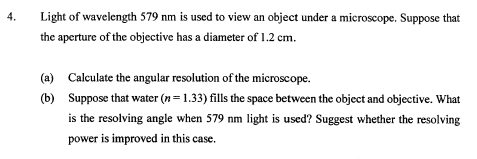# Angular resolution of microscope

## Homework Statementθ =1.22λ/d

## The Attempt at a Solution

for (a) θ =1.22λ/d
θ =1.22(579nm)/(1.2cm)[/B]
for the micorscope, the formula of angular resolution is different?
i find that NA=nsina (but how can i link with λ and d?)

The attempt at a solution
for (a) θ =1.22λ/d
θ =1.22(579nm)/(1.2cm)